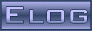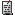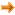DRS4 Forum
 DRS4 Discussion Forum Not logged inThu Apr 9 11:46:33 2015, Felix Bachmair, DRSBoard::SetTriggerSourceTue Apr 21 12:01:45 2015, Stefan Ritt, DRSBoard::SetTriggerSource
Message ID: 405     Entry time: Tue Apr 21 12:01:45 2015     In reply to: 402
 Author: Stefan Ritt Subject: DRSBoard::SetTriggerSource

Your first assumption is correct, e.g.

source = 00000000'00000001 = 0x0001 ==> CH1

source = 00010001'00000000 = 0x1100 ==> CH1 and EXT

So the lower byte is the "OR" block, and the upper byte is the "AND" block. Both blocks are combined via an "OR" so

source = 00011000'00000011 = 0x1803 is (EXT and CH4) OR (CH1 or CH2)

The "OR" combination between the two blocks is fixed in the firmware and cannot be changed without changing the firmware, but theoretically any logical combination between five inputs would be possible if you touch thr firmware.

/Stefan

 Felix Bachmair wrote: Hi I have a question about the function SetTriggerSource in the class DRSBoard (DRS.h/DRS.cpp) In the implementation there is the following comment: // Set trigger configuration // OR 0=CH1, 1=CH2, 2=CH3, 3=CH4, 4=EXT // AND 8=CH1, 9=CH2, 10=CH3, 11=CH4, 12=EXT   What does this exactly mean? I am assuming that this are the bits which are set? e.g source = 1 ==> CH1 source = 4352 = 0x1100 ==> CH1 and ext How is the AND/Or logic implemented? When i have: source = 0x1803 (bit 12,11,1,0) what is the right way to set the brackets to expalin the logic? (EXT and CH4 ) or CH2 or CH1 ?   Cheers Felix Bachmair ETH Zurich

ELOG V3.1.4-bcd7b50